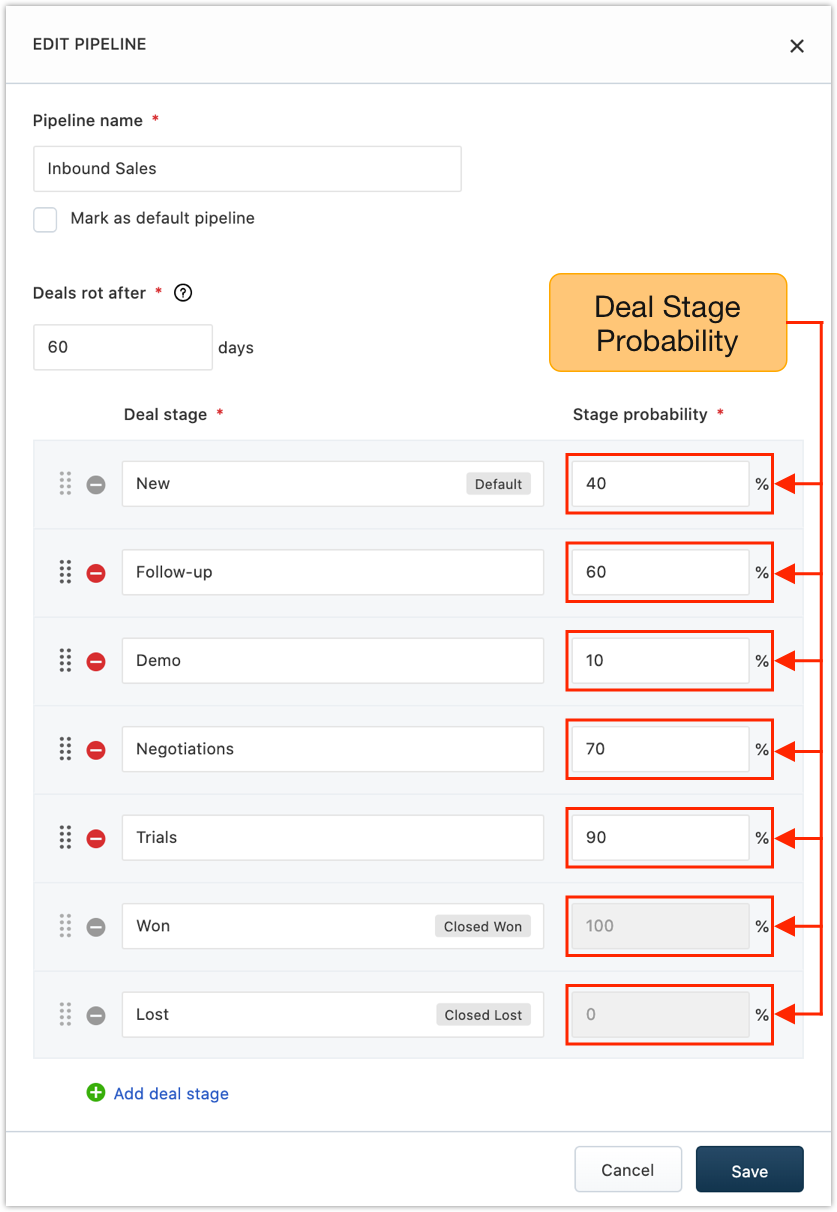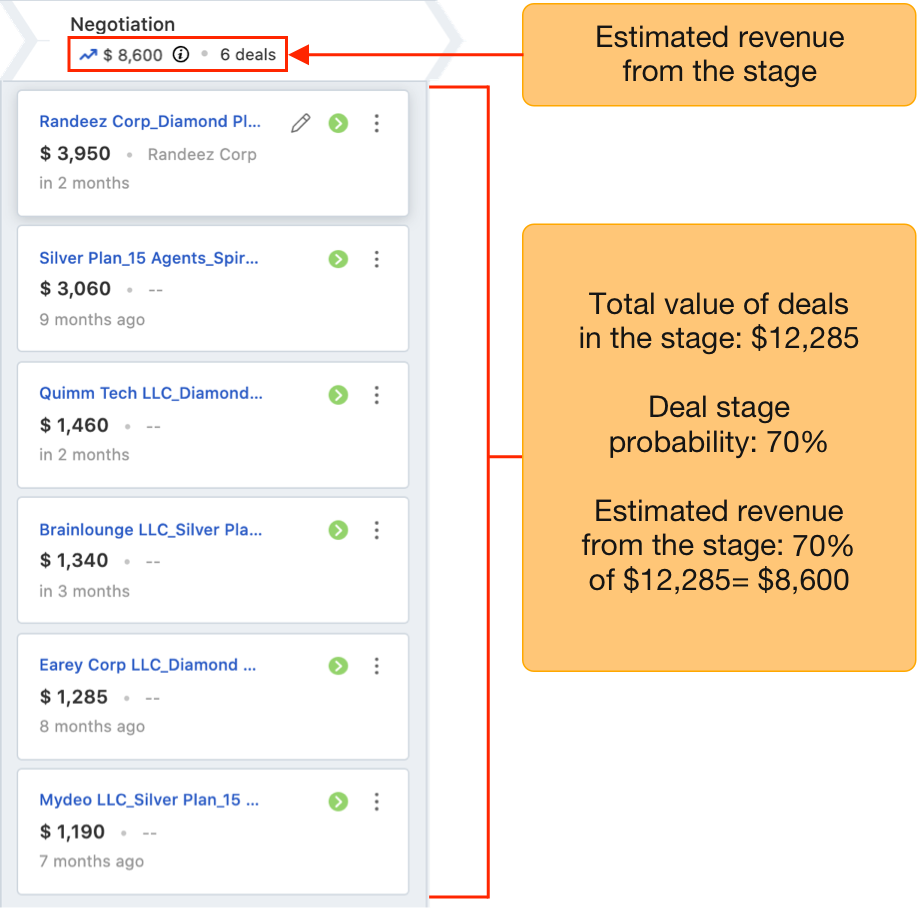Set an estimated probability against every deal stage to forecast expected sales based on the probability of deals in the pipeline.

How to set up weighted pipelines?

Here’s how:

1) Go to Admin Settings > CRM Modules and Automation > Pipelines and Goals > Pipelines.

2) Click onassociated with a pipeline. This opens the pipeline and its various deal stages.

`Note: You can also assign a rotting date for the pipeline while assigning probability for the stages. Know more about it here.`

3) Assign deal stage probability for each deal stage and click. The probability is a percentage operator and operates on the total deal value of the stage. Users can change the probability of deals manually if required.`Note: When deal stage probability is edited, the new percentage will only be reflected on new deals. The percentage of existing deals will not change.`

How to forecast sales based on these probabilities?

In any given deal stage, only a fraction of deals converts successfully. By factoring in this probability for every deal stage, revenue from individual stages can be estimated realistically.

For example, let’s consider a deal stage, ‘Negotiation.’ At this stage of the deal cycle, the probability of winning the deal is about 70%.

Now, let us consider that you have 6 deals in this stage, with each carrying values of \$3950, \$3060, \$1460, \$1340, \$1285, and \$1190, respectively.

The total deal value from this stage is \$12285.

However, the expected deal value from this stage will be calculated on the basis of the deal stage probability
(70%). Therefore, the expected revenue from this stage will be 70% of \$12285= \$8600